Smartick is an online platform for children to master math in only 15 minutes a day

Jul30

# Geometric Shapes: Characteristics of Triangles

In previous posts we explored geometric shapes such as the circle and the square. Now it is time to get to know the triangle better and understand its characteristics.

The triangle, as its name indicates, has three angles. It also has three sides. This makes it the geometric shape formed by the lowest number of sides and angles.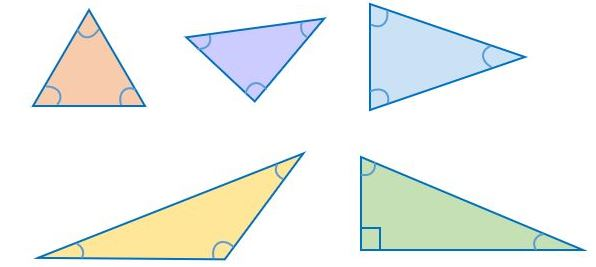As you can see, there are many different types of triangles, but the difference between them does not depend on their size or orientation but on the measurement of their angles. One important fact is that the sum of all three angles’ measurements is always 180º. This is a property that every triangle has.

We are going to review the most important types of triangles and their characteristics. If you need to review types of angles, you can take a look at previous posts.

### Classifying Triangles According to the Relationship Between their Angles:

#### Equilateral Triangle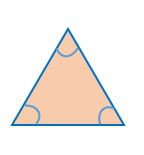This triangle’s name indicates that its three sides are equal in length. In equilateral triangles, all three angles are equal; each has the same measurement. If we divide 180º by the triangle’s number of sides to determine the three angles’ measurements, we will get 60º.  Each of the equilateral triangle’s three angles measure 60º.

#### Scalene Triangle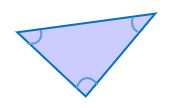This triangle is the opposite of the equilateral triangle. In scalene triangles, all of the angles are different; each one has a different measurement. This characteristic makes each of the triangle’s three sides have different measurements.

#### Isosceles Triangle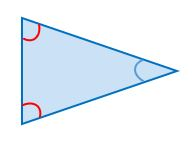Isosceles triangles have two equal angles and one that is different. This makes the triangles have two equal sides and one different side as well. The side that is different is located precisely between the equal angles.

### Classifying Triangles According to their Angle Type:

#### Acute Triangle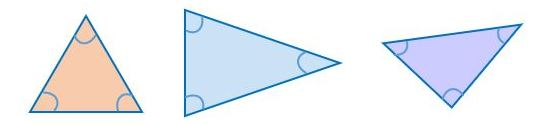All of the acute triangle’s angles are acute. The equilateral triangle is also acute since all of its angles measure 60º. Isosceles and scalene triangles can also be acute if all of their angles are acute.

#### Right Triangle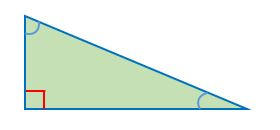There is one right angle in right triangles. There can only be one right angle in this type of triangle. The right angle measures 90º and the triangle has three angles that add up to 180º, therefore the other two angles are acute and add up to 90º. A right triangle can be isosceles if its two acute angles both measure 45º, and if all angles have different measurements, the triangle will be scalene.

#### Obtuse Triangle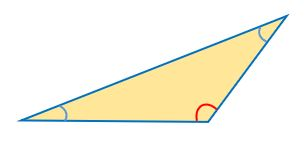In obtuse triangles, one angle is obtuse. The other two are acute. If the acute angles are equal, the obtuse triangle will also be isosceles. If all of the angles are different, the triangle will be scalene.

We have seen that triangles are the geometric shapes with the lowest number of angles and sides. We have also seen that there are different classifications of triangles depending on their angles’ measurements and that within these classifications, different types are combinable with each other. For example, we can have scalene right-angled triangles or obtuse isosceles triangles.